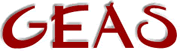[ < ] [ > ] [ << ] [ Up ] [ >> ] [Top] [Contents] [Index] [ ? ]

### 2.2.9 Array & Mapping references

In comp sci terms, arrays and mappings are used as reference by pointer and the other types as reference by value. This means that arrays and mappings, unlike other variables, aren't copied every time they are moved around. Instead, what is moved is a reference to the original array or mapping. What does this mean then?

Well... simply this:

 ```object *arr, *copy_arr; arr = ({ 1, 2, 3, 4 }); // An array copy_arr = arr; // Assume (wrongly) that a copy_arr becomes // a copy of arr. // Change the first value (1) into 5. copy_arr = 5; ```

... Now... this far down the code it's logical to assume that the first value of `copy_arr` is 5 while the first value or `arr` is 1. That's not so however, because what got copied into `copy_arr` was not the array itself, but a reference to the same array as `arr`. This means that your operation later where you changed an element, changed that element in the original array which both variables refer to. `copy_arr` and `arr` will both seem to have changed, while in fact it was only the original array that both referred to that changed.

Exactly the same thing will happen if you use mappings since they work the same way in this respect.

So... how do you get around this then? I mean... most times you really want to work on a copy and not the original array or mapping. The solution is very simple actually. You just make sure that the copy is created from another array or mapping instead.

 ``` _ This is just an empty array / copy_arr = ({ }) + arr; \_ This is the one we want to make unique ```

In this example `copy_arr` becomes the sum of the empty array and the `arr` array created as an entirely new array. This leaves the original unchanged, just as we wanted. You can do exactly the same thing with mappings. It doesn't matter if you add the empty array or mapping first or last, just as long as you do it.

 [ < ] [ > ] [ << ] [ Up ] [ >> ] [Top] [Contents] [Index] [ ? ]

This document was generated by Ronny Wikh on July, 8 2003 using texi2html